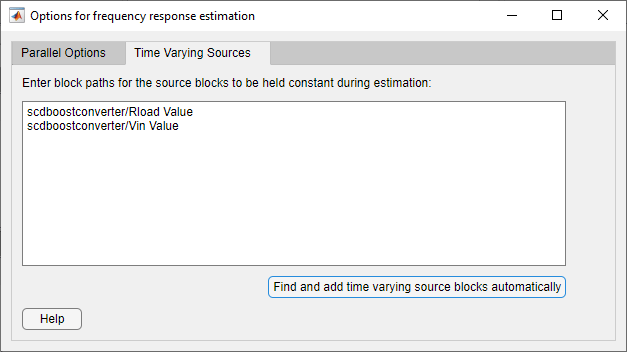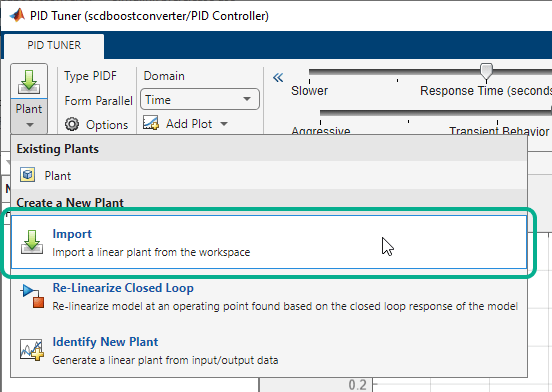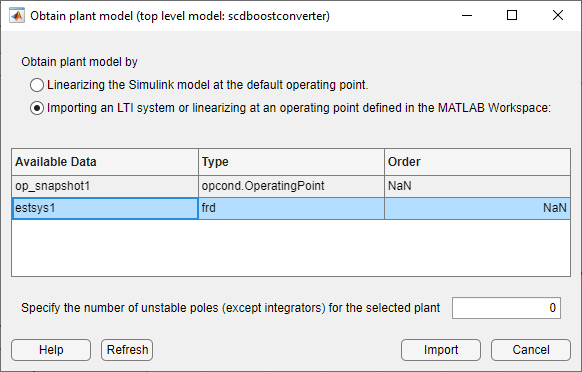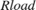# Design Controller for Power Electronics Model Using Frequency Response Data

This example shows how to design a PID controller for a power electronics system modeled in Simulink® using Simscape™ Electrical™ components.

Typically, power electronics systems cannot be linearized because they use high-frequency switching components, such as pulse-width modulation (PWM) generators. However, most Simulink® Control Design™ PID tuning tools design PID gains based on a linearized plant model. To obtain such a model for a power electronics model that cannot be linearized, you can:

### Boost Converter Model

This example uses a boost converter model as an example of a power electronics system. A boost converter circuit converts one DC voltage to another, typically higher, DC voltage by controlled chopping or switching of the source voltage.

```mdl = 'scdboostconverter'; open_system(mdl) ```In this model, a MOSFET driven by a pulse-width modulation (PWM) signal is used for switching. The output voltageshould be regulated to the reference value. A digital PID controller adjusts the PWM duty cycle,, based on the voltage error signal. For this example, you estimate the frequency response from the PWM duty cycle to the load voltage.

Simscape Electrical software contains predefined blocks for many power electronics systems. This model contains a variant subsystem with two versions of the boost converter model:

• Boost converter circuit constructed using electrical power components. The parameters of the circuit components are based on .

• Boost converter block configured to have the same parameters as the boost converter circuit. For more information on this block, see Boost Converter (Simscape Electrical).

### Find Model Operating Point

To design a controller for the boost converter, you must first determine the steady-state operating point at which you want the converter to operate. For more information on finding operating points, see Find Steady-State Operating Points for Simscape Models. For this example, use an operating point estimated from a simulation snapshot.

To find the operating point, use the Model Linearizer. To open Model Linearizer, in the Simulink model window, on the Apps tab, click Model Linearizer.

In the Model Linearizer, on the Linear Analysis tab, in the Operating Point drop-down list, select Take Simulation Snapshot.In the Enter snapshot times to linearize dialog box, in the Simulation snapshot times field, enter `0.045`, which is enough time for the closed-loop system to reach steady state.Click Take Snapshots.

The software simulates the model and creates an operating point that contains the input and state values of the model at the specified snapshot time. This operating point, `op_snapshot1`, is added to the Linear Analysis Workspace.To initialize the model with the computed operating point, double-click `op_snapshot1`.In the Edit dialog box, click Initialize model.In the Initialize Model dialog box, select MATLAB Workspace, and click OK. The software exports the operating point to the MATLAB® workspace and initializes the model with the inputs and states in the operating point.

### Collect Frequency Response Data

Before collecting frequency response data, you must first specify the portion of the model for which you want to find the frequency response. For this example, the model contains open-loop input and output linear analysis points at the output and input of the PID controller block.

To collect frequency response data, you must also specify an input signal. For this example, use a fixed-step sinestream signal. For more information on defining sinestream input signals, see Sinestream Input Signals.

On the Estimation tab, in the Input Signal drop-down list, click Fixed Sample Time Sinestream.In the Specify fixed sample time dialog box, specify a Sample time of `5e-6` seconds. The sample time of the Sinestream input signal must match the sample time at the input linear analysis point.Click OK.

In the Create sinestream input with fixed sample time dialog box, configure the parameters of the sinestream signal.

Specify the frequency units for estimation. In the Frequency units drop-down list, select `Hz`.

For this example, the frequency response estimation can either use one simulation per frequency or one simulation for all frequencies. In the Simulation order drop-down list, select the default option Single simulation for all frequencies. If you have Parallel Computing Toolbox™ software, you can speed up the frequency response estimation by choosing One frequency per simulation and enabling the parallel pool for estimation. To enable the parallel pool, on the Estimation tab, click More Options, then in the dialog box, select Use parallel pool during estimation.

To specify the frequencies at which to estimate the plant response, click the `+` icon.In the Add frequencies dialog box, specify `15` logarithmically-spaced frequencies ranging from `50 Hz` to `5 kHz`.Click OK.

To ensure the system is properly excited, set the amplitude at all frequencies. If the input amplitude is too large, the boost converter will operate in discontinuous-current mode. If the input amplitude is too small, the sinestream will be indistinguishable from ripples in the power electronics circuits. Both situations produce inaccurate frequency response estimation results.

To set the amplitude, first select all the frequencies in the plot area. Then, in the Amplitude field, type `0.01`.

Leave all other sinestream settings at their default values.To create the sinestream signal, click OK.

The model has time-varying line and load disturbances modeled as step functions that will interfere with the frequency response estimation. To hold these disturbances constant during the simulation, click More Options. Then, in the Options for frequency response estimation dialog box, on the Time Varying Sources tab, click Find and add time varying source blocks automatically.To estimate and plot the frequency response, on the Estimation tab, click Bode.The software estimates the frequency response and displays the result in Bode Plot 1. The frequency response is plotted using discrete points and shows the peak response between 1200 and 1600 rad/s.

To tune your PID controller, you must export the frequency response to the MATLAB workspace. In the Data Browser, drag `estsys1` from the Linear Analysis Workspace to the MATLAB Workspace.### Specify Controller Structure

Before tuning a PID Controller block using PID Tuner, you must first specify your controller structure. To do so, double-click the PID Controller block. Then, specify the following controller parameters:

• Controller

• Form

• Time domain

• Discrete-time settings

• Other settings, such as the controller initial conditions, output saturation levels, and anti-windup configuration

For this example, use the current controller configuration; that is, a discrete-time parallel-form PID controller without anti-windup.

Using the PID Tuner, you can tune the parameters of the following controller blocks:

If your model uses the Simscape Electrical Discrete PI Controller (Simscape Electrical) block or Discrete PI Controller with Integral Anti-Windup (Simscape Electrical) block, you must replace this block with a PID Controller block before tuning.

### Tune Controller

To open the PID Tuner, click Tune. When PID Tuner first opens, it attempts to linearize the model. Due to the PWM components, the model analytically linearizes to zero.

For this example, you tune the controller using the estimated frequency response data as your plant model. To import the frequency response data, on the PID Tuner tab, click Plant, and then, under Create a New Plant, click Import.In the Obtain plant model dialog box, select Importing an LTI System, and in the table, select `estsys1`.Click Import.

Since you are using an estimated frequency response, PID Tuner cannot plot a step response. To view the frequency response, click Add Plot, and under Bode, click `Open-loop`.Close the Step Plot document.

The Bode plot shows a block response (dashed line) and a tuned response (solid line). The block response is the open-loop response for the current PID gains in the PID Controller block. The tuned response is the open-loop response using the tuned PID gains in PID Tuner.To tune the controller in terms of bandwidth and phase margin, design the controller in the frequency domain. In the Domain drop-down list, select `Frequency`.

For this example, set the Bandwidth and Phase Margin to 9425 rad/s (1.5 kHz) and 60 deg, respectively, according to the design criteria specified in .

PID Tuner selects controller parameters that meet these design specifications.To view the tuned controller parameters and performance metrics, including the gain and phase margins, click Show Parameters. The tuned result has an infinite gain margin and 65 deg phase margin at about 9425 rad/s.To update the PID Controller block with the tuned gains, click Update Block.

### Validate Controller

You can examine the tuned controller performance using a simulation with line and load disturbances. To examine the controller dynamic performance, the Simulink model uses the following disturbances:

• Line disturbance at t = 0.075 sec, which increases the input voltage,, from 5V to 10V.

• Load disturbance at t = 0.1 sec, which increases the load resistance,from 3 ohms to 6 ohms.

Simulate the model.The controller rejects the line and load disturbances well.

### References

 Lee, S. W. "Practical Feedback Loop Analysis for Voltage-Mode Boost Converter." Application Report No. SLVA633. Texas Instruments. January 2014. www.ti.com/lit/an/slva633/slva633.pdf